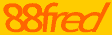# Introduction to Time Value of Money

Written by Clayton Reeves for Gaebler Ventures

Looking for a primer on the time value of money? You're in the right spot! Some big corporations still have a hard time getting a grasp on this concept, so if your company can implement it into your planning process, you will have a leg up on the big guys.

Time value of money is a concept that is vital to gaining a better understand of financial concepts.

In small businesses, money can be received at a variety of times for services rendered. There may be n/2 or n/30 arrangements that will let a payment be delayed by a month. A business must consider the pros and cons to letting customers delay payment, as these decisions can impact earnings

Money is more valuable when received today, as opposed to tomorrow. This is because if the money is received today, it can be invested overnight and earns a small but tangible return. Present value and future value are two terms that will be discussed frequently when describing the time value of money. This is because they are the two main drivers of the theory.

Present value is how much a future cash flow is worth today, given a discount factor. Future value is the exact opposite; how much is money today worth at some point in the future?

These two tools can help businesses decide whether or not to pay in cash or accept financing deals. The time value of money is often why paying in full can get you a better deal on anything from supplies to your car insurance. I'll go over briefly how present and future value factors work

Future value is much easier to understand at first than present value. If an investor buys a \$100 asset and expects to receive 5% on that asset, it will be worth \$105 dollars after one year. Here is the equation:

FV = 100(1.05) or FV = PV(1 + i), where FV is future value, PV is present value, and i is the percentage return expected to be earned on that investment. Another example would be FV = 1000(1.12) = 1120

This is the equation for future value. If you wanted to get the future value after two years, you would simply multiply the initial investment by 1.05 twice; one for the first year, and another for the second year. In order to get the return for two years, you simply raise the interest to the power of n = the number of years you have the investment. For instance, if the investor invested that same 100 dollars for two years instead of one, it would look like this.

FV = 100(1.05)(1.05) = 110.25 or FV = 100(1.05)^2

This gives you the future value after two years. Now, in order to get the present value you simply use algebra to switch around the equation. Instead of multiplying the PV by (1+i), you divide the FV by (1+i). This would give you: 105/(1.05) = PV

These are generally pretty simply concepts, but practicing with some numbers will give you a better idea of how money can grow when invested. Money is always more valuable today than it will be tomorrow, all other things equal. Remember this when deciding on how to structure payment terms for your business.

When he's not playing racquetball or studying for a class, Clayton Reeves enjoys writing articles about entrepreneurship. He is currently an MBA student at the University of Missouri with a concentration in Economics and Finance.

## Conversation Board

How do you use the time value of money to make better decisions?